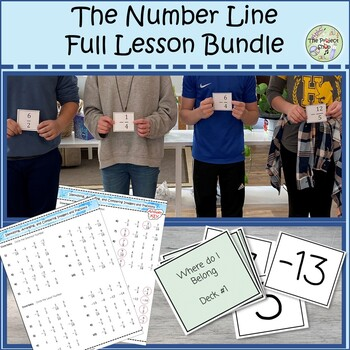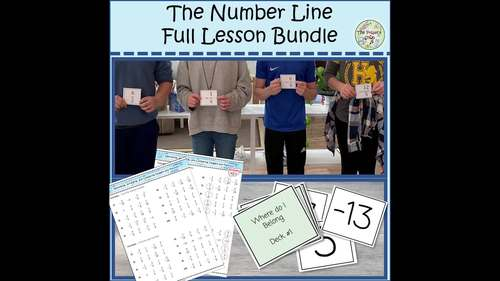# Number Line Lesson Bundle--Games, Hands-on Materials, Fractions & IntegersSubject
Resource Type
File Type

Zip

(5 MB|271 pages)
Standards
3 Products in this Bundle
3 products
1. Learning fractions can be hard enough without half of them being negative! In this game, students work together to order themselves as positive & negative fractions & integers—quickly learning the symmetry of the number line! In this resource you get:Three Scaffolded Decks of CardsDeck #1: I
2. Students are often confused about which direction to go when performing operations on the number line. Even more challenging is adding and subtracting negatives! This movement game clears up the confusion!In this fun and interactive movement game, students stand on their number and are given a set o
3. Are your students struggling with making the leap from positive and negative integers to positive and negative fractions? This lesson anchors numbers to the number line with hands-on materials and reflection questions in a way that sets students up for success!In this lesson, students will learn… Ho
• Bundle Description
• StandardsNEW

Are your students struggling with making the leap from positive and negative integers to positive and negative fractions? This lesson bundle anchors numbers to the number line with hands-on materials, movement, games, and reflection questions in a way that sets students up for success!

In this lesson students will learn…

• How to compare and order both positive and negative fractions and integers
• How to think about integers while ordering and comparing fractions
• How to mentally convert to mixed numbers while ordering fractions

In this lesson students will understand…

• The symmetry of the number line
• Opposites of numbers and operations
• That fractions belong on the same number line as integers

This lesson bundle includes…

• A Pacing and Lesson Plan Guide for a 2-3-day lesson

• Two Daily Warmups in a PowerPoint File

• Number Line Printable Materials—independent instructions for these printable in-class or take-home materials

• 1 Group Game—The Human Number Line, which can also be turned into a tabletop game with the hands-on materials in this lesson

• 4 Number Line Card Games—Where do I Belong, Integer and Fractions Dominoes, Integer and Fractions Puzzle, and War!

• Worksheets and Homework Pages—practice problems and reflection questions for each day get students thinking about the symmetry of the number line

• Editable Quiz

Don’t forget to follow me to be notified of new products and dollar deal days!

Enjoy!

Apply properties of operations as strategies to add and subtract rational numbers.
Understand subtraction of rational numbers as adding the additive inverse, 𝘱 – 𝘲 = 𝘱 + (–𝘲). Show that the distance between two rational numbers on the number line is the absolute value of their difference, and apply this principle in real-world contexts.
Apply and extend previous understandings of addition and subtraction to add and subtract rational numbers; represent addition and subtraction on a horizontal or vertical number line diagram.
Total Pages
271 pages
Included
Teaching Duration
3 days
Report this Resource to TpT
Reported resources will be reviewed by our team. Report this resource to let us know if this resource violates TpT’s content guidelines.